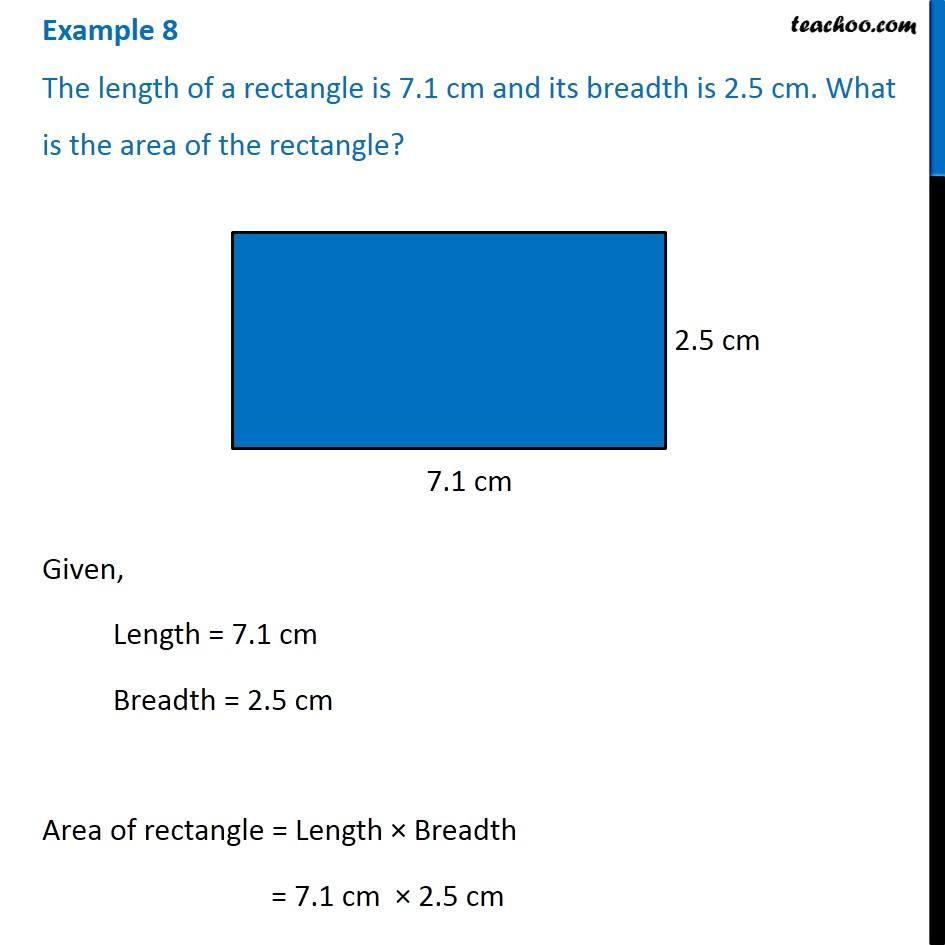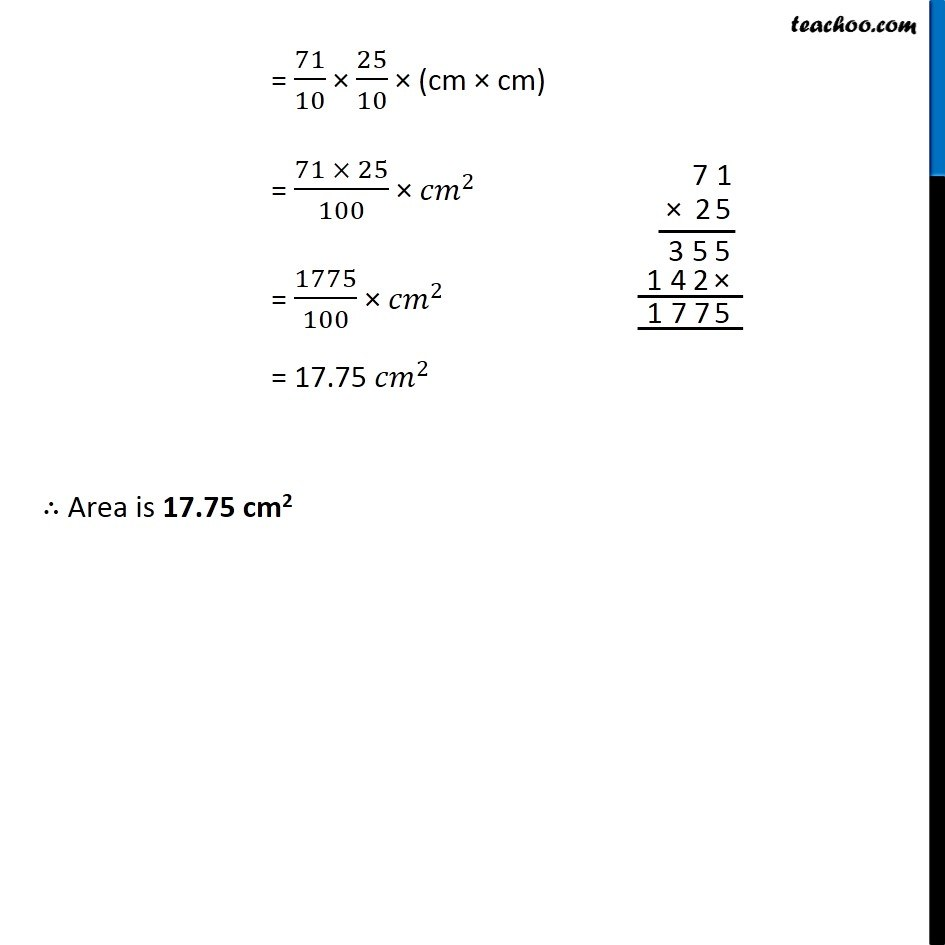Examples

Chapter 2 Class 7 Fractions and Decimals
Serial order wiseLearn in your speed, with individual attention - Teachoo Maths 1-on-1 Class

### Transcript

Example 4 The length of a rectangle is 7.1 cm and its breadth is 2.5 cm. What is the area of the rectangle?Given, Length = 7.1 cm Breadth = 2.5 cm Area of rectangle = Length × Breadth = 7.1 cm × 2.5 cm = 71/10 × 25/10 × (cm × cm) = (71 × 25)/100 × 〖𝑐𝑚〗^2 = 1775/100 × 〖𝑐𝑚〗^2 = 17.75 〖𝑐𝑚〗^2 ∴ Area is 17.75 cm2